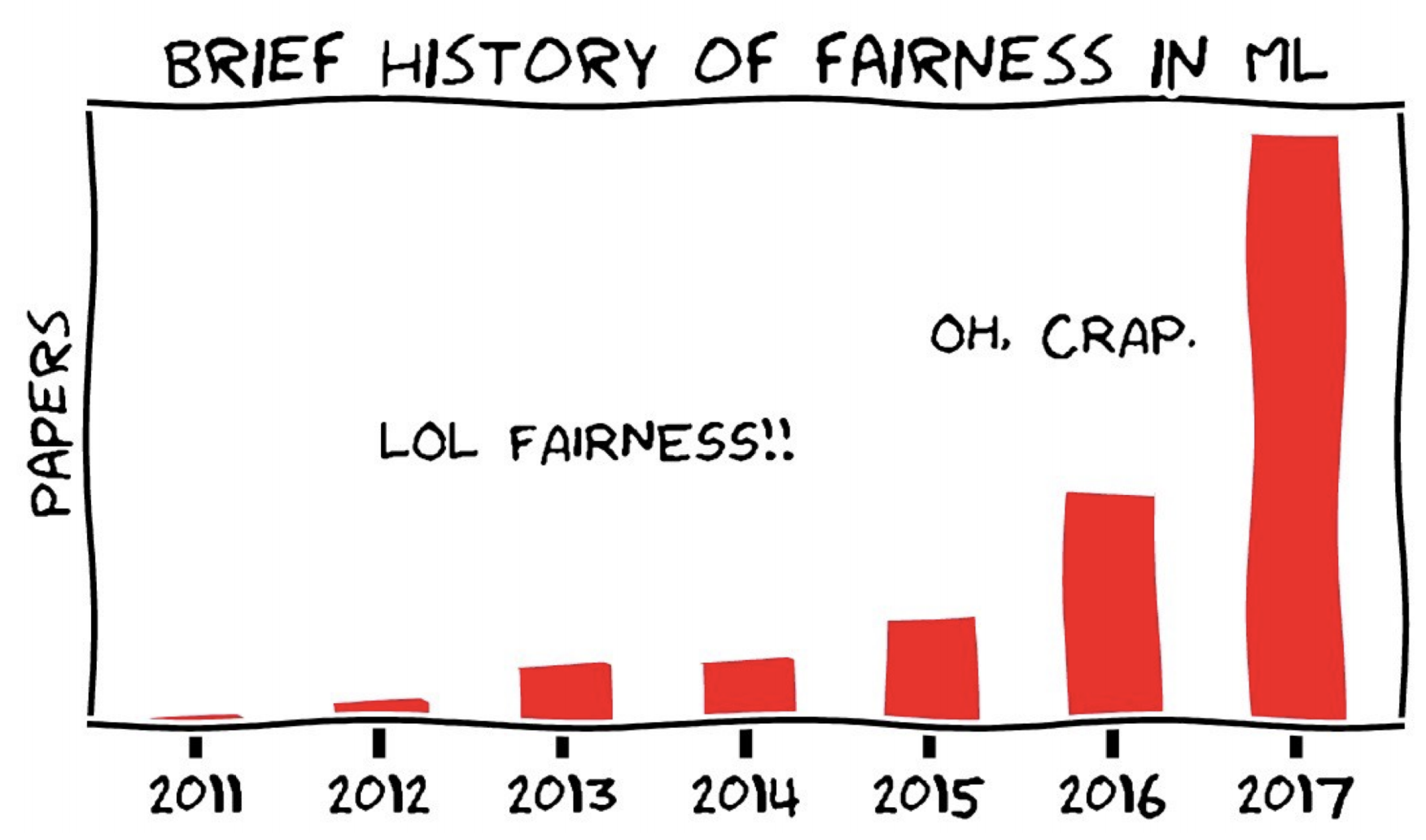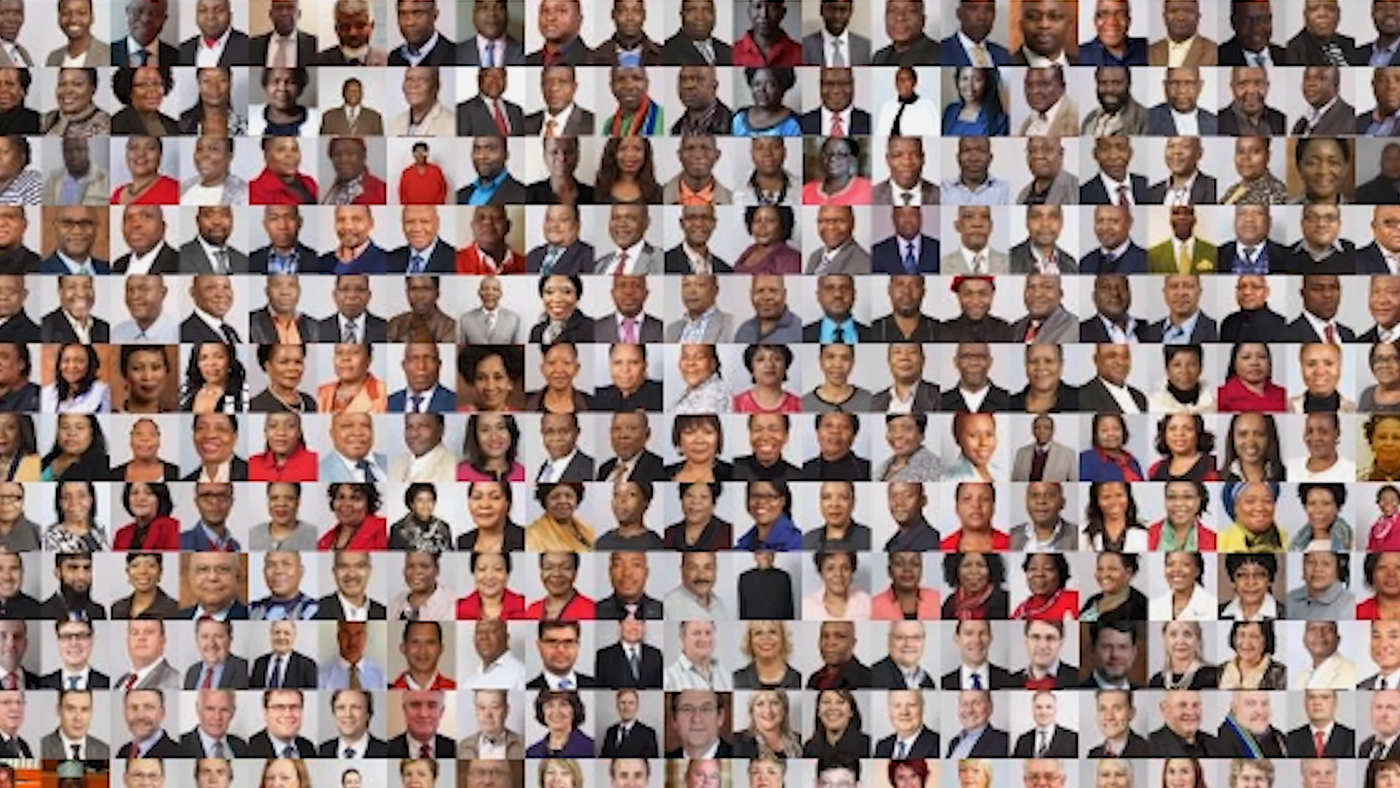# Fairness in Artificial Intelligence

Artificial Intelligence often gives the impression that it is objective and "fair". However, algorithms are made by humans and trained by data which may be biased. There are several examples of deployed AI algorithms that have been shown to make decisions that were biased based on gender, race or other protected demographics — even when there was no intention for it.

These examples have also led to a necessary research into a growing field of algorithmic fairness. How can we demonstrate, or prove, that an algorithm is behaving in a way that we think is appropriate? What is fair? Clearly these are complex questions and are deserving of a complete conversation. This example is simple for the purpose of giving an introduction to the topic.ML stands for Machine Learning. Solon Barocas and Moritz Hardt, "Fairness in Machine Learning", NeurIPS 2017

## What is Fairness?

An artificial intelligence algorithm is going to be used to make a binary prediction ($G$ for guess) for whether a person will repay a loan. The question has come up: is the algorithm "fair" with respect to a binary protected demographic ($D$ for demographic)? To answer this question we are going to analyze predictions the algorithm made on historical data. We are then going to compare the predictions to the true outcome ($T$ for truth). Consider the following joint probability table from the history of the algorithm’s predictions:

$D=0$

$G=0$ $G=1$
$T=0$ 0.21 0.32
$T=1$ 0.07 0.28
$D=1$

$G=0$ $G=1$
$T=0$ 0.01 0.01
$T=1$ 0.02 0.08

Recall that cell $D=i,G=j,T=k$ contains the probability $\P(D=i,G=j,T=k)$. A joint probability table gives the probability of all combination of events. Recall that since each cell is mutually exclusive, the $\sum_i \sum_j \sum_k \P(D=i,G=j,T=k) = 1$. Note that this assumption of mutual exclusion could be problematic for demographic variables (some people are mixed ethnicity, etc) which gives you a hint that we are just scratching the surface in our conversation about fairness. Let's use this joint probability to learn about some of the common definitions of fairness.

Practice with joint marginalization
What is $\p(D=0)$? What is $\p(D=1)$?
Probabilities with assignments to a subset of the random variables in the joint distribution can be calculated by a process called marginalization: sum the probability from all cells where that assignment is true.

\begin{align} \p(D=1) &= \sum_{j \in \{0, 1\}} \sum_{k \in \{0, 1\}} \p(D= 1, G= j, T = k)\\ &= 0.01 + 0.01 + 0.02 + 0.08 = 0.12 \end{align} \begin{align} \p(D=0) &= \sum_{j \in \{0, 1\}} \sum_{k \in \{0, 1\}} \p(D= 0, G= j, T = k)\\ &= 0.21 + 0.32 + 0.07 + 0.28 = 0.88 \end{align}

Note that $\p(D=0) + \p(D=1) = 1$. That implies that the demographics are mututally exclusive.

Fairness definition #1: Parity
An algorithm satisfies “parity” if the probability that the algorithm makes a positive prediction ($G$ = 1) is the same regardless of being conditioned on demographic variable.

Does this algorithm satisfy parity?

\begin{align} P(G=1|D=1) &= \frac{P(G = 1, D = 1)}{P(D=1)} && \text{Cond. Prob.}\\ &= \frac{P(G = 1, D = 1, T= 0) + P(G=1, D = 1, T=1)}{P(D=1)} && \text{Prob or}\\ &= \frac{0.01 + 0.08}{0.12} = 0.75 && \text{From joint} \end{align} \begin{align} P(G=1|D=0) &= \frac{P(G = 1, D = 0)}{P(D=0)} && \text{Cond. Prob.}\\ &= \frac{P(G = 1, D = 0, T= 0) + P(G=1, D = 0, T=1)}{P(D=0)} && \text{Prob or}\\ &= \frac{0.32 + 0.28}{0.88} \approx 0.68 && \text{From joint} \end{align}

No. Since $P(G=1|D=1) \neq P(G=1|D=0)$ this algorithm does not satisfy parity. It is more likely to guess 1 when the demographic indicator is 1.

Fairness definition #2: Calibration
An algorithm satisfies “calibration” if the probability that the algorithm is correct ($G=T$) is the same regardless of demographics.

Does this algorithm satisfy calibration?

The algorithm satisfies calibration if $P(G = T | D = 0) = P(G = T | D = 1)$ \begin{align} P(G = T | D = 0) &= P(G = 1, T = 1 | D = 0) + P(G = 0, T = 0 | D = 0)\\ &= \frac{0.28 + 0.21}{0.88} \approx 0.56 \\ P(G = T | D = 1) &= P(G = 1, T = 1 | D = 1) + P(G = 0, T = 0 | D = 1)\\ &= \frac{0.08 + 0.01}{0.12} = 0.75 \end{align} No: $P(G = T | D = 0) \neq P(G = T | D = 1)$

Fairness definition #3: Equality of Odds
An algorithm satisfies "equality of odds" if the probability that the algorithm predicts a positive outcome ($G=1$) is the same regardless of demographics given that the outcome will occur ($T=1$).

Does this algorithm satisfy equality of odds?

The algorithm satisfies equality of odds if $P(G = 1 | D = 0, T = 1) = P(G = 1 | D = 1, T = 1)$ \begin{align*} P(G = 1 | D = 1, T = 1) &= \frac{P(G = 1 , D = 1, T = 1)}{P(D = 1, T = 1)}\\ &= \frac{0.08}{0.08 + 0.02} = 0.8 \\ P(G = 1 | D = 0, T = 1) &= \frac{P(G = 1 , D = 0, T = 1)}{P(D = 0, T = 1)}\\ &= \frac{0.28}{0.28 + 0.07} = 0.8 \end{align*} Yes: $P(G = 1 | D = 0, T = 1) = P(G = 1 | D = 1, T = 1)$

Which of these definitions seems right to you? It turns out, it can actually be proven that these three cannot be jointly optimized, and this is called the Impossibility Theorem of Machine Fairness. In other words, any AI system we build will necessarily violate some notion of fairness. For a deeper treatment of the subject, here is a useful summary of the latest research Pessach et al. Algorithmic Fairness.

In 2018, Joy Buolamwini and Timnit Gebru had a breakthrough result called "gender shades" published in the first conference on Fairness, Accountability and Transparency in ML . They showed that facial recognition algorithms, which had been deployed to be used by Facebook, IBM and Microsoft, were substantially better at making predicitons (in this case classifying gender) when looking at lighter skinned men than darker skinned women. Their work exposed several shortcomings in production AI: biased datasets, optimizing for average accuracy (which means that the majority demographic gets most weight) lack of awareness of intersectionality, and more. Let's take a look at some of their results.Figure by Joy Buolamwini and Timnit Gebru. Facial recognition algorithms perform very differently depending on who they are looking at. 

Timnit and Joy looked at three classifiers trained to predict gender, and computed several statistics. Let's take a look at one statistic, accuracy, for one of the facial recognition classifiers, IBMs:

Women Men Darker Lighter
Accuracy 79.7 94.4 77.6 96.8

Using the language of fairness, accuracy measures $\p(G=T)$. The cell in the table above under "Women" says the accuracy when looking at photos of women $\p(G=T|D = \text{Women})$. It is easy to show that these production level systems are terribly "uncalibrated": $$\p(G=T|D = \text{Woman}) \neq \p(G=T|D = \text{Man})$$ $$\p(G=T|D = \text{Lighter}) \neq \p(G=T|D = \text{Darker})$$

Why should we care about calibration and not the other definitions of fairness? In this case the classifier was making a prediction of gender where a positive prediction (say predicting women) doesn't have a directly associated reward as in our above example, where we were predicting if someone should receive a loan. As such the most salient idea is: is the algorithm just as accurate for different genders (calibration)?

The lack of calibration between men/women and lighter/darker skinned photos is an issue. What Joy and Timnit showed next was that the problem becomes even worse when you look at intersectional demographics.

Darker Men Darker Women Lighter Men Lighter Women
Accuracy 88.0 65.3 99.7 92.9

If the algorithms were "fair" according to the calibration you would expect the accuracy to be the same regardless of demographics. Instead there is almost a 34.2 percentage point difference! $\p(G=T|D = \text{Darker Woman})$ = 65.3 compared to $\p(G=T|D = \text{Ligher Man}) = 99.7$